AZ Math Tutors is offering head start courses to make  your next school year an easier one. As always, AZ  Math Tutors customizes the program to suit your individual needs.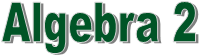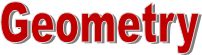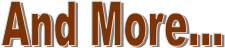Learn how to solve and graph:  Systems of equations, systems of inequalities, and quadratic equations. Simplify complicated exponential fractions, polynomial fractions, and radical fractions.
 Learn how to discover the Side and angle measures of triangles, and quadrilaterals inside and outside of circles. Learn how to discover area, perimeter, and circumference of various two dimensional shapes.   Learn surface area and volume of 3D shapes such as prims and pyramids.
 Learn how to Work with matrices; factor, solve and graph third degree polynomials; Solve    systems with three or more equations or inequalities. Work with imaginary numbers, logarithms and e.  Graph circles, ellipses and hyperbolas.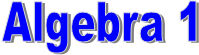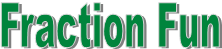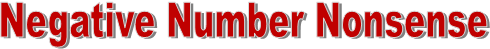This program is designed to eliminate fraction fear and increase student potential. Improve fraction skills in an non-stressful  environment.  Practice reducing, adding, subtracting, multiplying, dividing fractions and more, while still managing to smile.
 Understand how to work with integers.  Gain confidence answering math problems  that involve negative numbers.  Prevent Non-mastery of this topic from lowering high school math grades.
 QUESTIONS?  Ready to reserve your place?     Call or EMAIL NOW  973-722-6284
 Want private lessons?    Have friends that you would want to group with?   Want to join a group being formed here?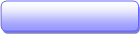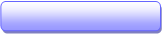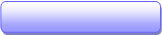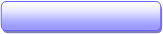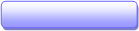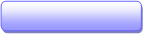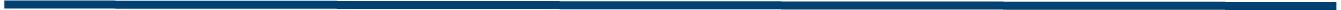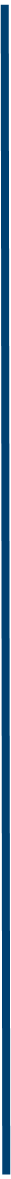AZ Math Tutors serves Morris county including Morristown, Morris Plains, Randolph, Denville, Parsippany-Troy,  Rockaway, Roxbury, Mt. Olive, and Chester